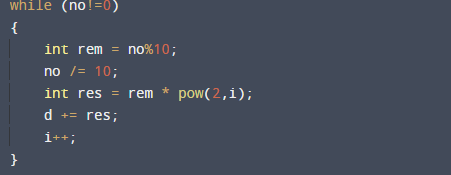# C++ Program to Convert Binary to Decimal

In this tutorial you will learn about the C++ Program to Convert Binary to Decimal and its application with practical example.

In this tutorial, we will create a c++ program to covert Binary to Decimal using C++ programming language.

## Prerequisites

Before starting with this tutorial we assume that you are best aware of the following C programming topics:

• Basic input/output Functions.
• Basic c++ programming.
• C++ Functions.
• User-defined Functions in C++.
• C++ Recursion.
• if…else statement.
• Loops.

## What Is Binary Number?

A number system in which numbers are represented by using only two digits (0 and 1) with a base 2 is called a binary number system.In the concept of converting a number from binary to a decimal number , extract all the digits of the binary number (starting from right of binary number )and multiply the each digits with the base(power of 2) lastly add each  number.As shown below.

Example: 1111
Decimal number =1*(2^3) + 1*(2^2) + 1*(2^1) + 1*(2^0)=8 + 4 +0+1 = 15.

## C++ Program to Convert Binary to Decimal.

In this program we will covert Binary to Decimal using user define function. We would first declared and initialized the required variables. Next, we would prompt user to input the number . Later we will covert specified number fro Binary to Decimal.

## OutputIn the above program, we have first declared and initialized a set variables required in the program.

• num = it will hold given binary number.
• BD =  User define function.
• no= catch value passing through function calling.
• i= for iteration.
• rem= hold the remainder.
• d= hold the decimal value.

In the next statement user will be prompted to enter a Binary number which will be pass through BD(num) function as a parameter. Now within the function using while loop digits of binary are extracted one by one starting from right as shown in below image.then extracted digits with remainders of the number are multiplied with powers of 2.
i.e. power of 2.   res = rem*pow(2,i) .
using inbuilt power function.
After multiplying each digit  results  added and stored in variable.
d(decimal) :=> d += res.
This process continues until while condition false ,and we finally we will print the result in output.

In this tutorial we have learn about the C++ Program to Convert Binary to Decimal and its application with practical example. I hope you will like this tutorial.